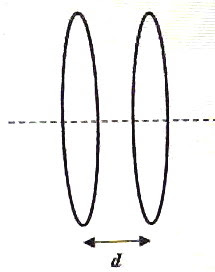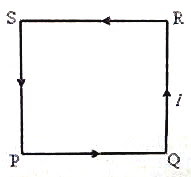## Pages

`“Life is like riding a bicycle.  To keep your balance you must keep moving.”–Albert Einstein`

## Thursday, March 25, 2010

### Multiple Choice Practice Questions on Magnetic Fields for AP Physics B & C

Equations to be remembered in the section ‘magnetic fields’ were discussed in the post dated 1st February 2008, followed by some multiple choice practice questions in the post dated 2nd February 2008. A free response practice question for AP Physics C aspirants was discussed in the post dated 9th February 2008, followed by another free response practice question of common interest to AP Physics B as well as AP Physics C aspirants. You can access all these posts by clicking on the label ‘magnetic field’ below this post.

Today we will discuss a few more multiple choice questions (for practice) on magnetic fields:

(1) Electrons and protons of the same momentum enter normally into a uniform magnetic field of flux density B. Mass of the electron is me and the mass of the proton is mp. If Re and Rp represent the radii of the paths of the electron and the proton respectively, then the ratio Re/Rp is

(a) mp/me

(b) me/mp

(c) (mp/me)1/2

(d) (me/mp)1/2

(e) 1

In the case of a particle of mass m and charge q projected with speed v perpendicular to a magnetic field of flux density B, the magnetic force of magnitude qvB supplies the centripetal force required for the circular motion of the particle within the field so that we have

qvB = mv2/R where R is the radius of the path.

Therefore, R = mv/qB

The electron and proton have the same quantity of charge (even though the signs are opposite). The momentum mv is given as the same in the question. Therefore they move along circular paths of the same radius so that the ratio Re/Rp = 1 [Option (e)].

(2) An particle of mass m and charge q is projected perpendicular to crossed static electric and magnetic fields. The magnetic field has magnitude k tesla while the electric field has magnitude 2k newton per coulomb. The kinetic energy of the electron is found to increase from 2.5 electron volt to 4 electron volt. How much energy is supplied (to the particle) by the magnetic field?

(a) 4 eV

(b) 1.5 eV

(c) 1.0 eV

(d) 0.5 eV

(e) zero

Even though there is a magnetic force on a charged particle moving through a magnetic field, the direction of this force is always perpendicular to the direction of the displacement of the particle. Therefore the work done by the magnetic field on the charged particle is zero. In other words, the energy supplied (to the particle) by the magnetic field is zero. [Option (e)].(3) The attractive force between two identical coaxial circular coils separated by a small distance d (fig.) and carrying the same current I is F. If the separation is halved and the current in both coils reversed and doubled, the force becomes

(a) F

(b) F

(c) 4F

(d) 8F

(e) – 8F

Two identical coils separated by a small distance can be treated as two parallel straight wires so that the magnetic force between them is directly proportional to the product of the currents in them and inversely proportional to the separation between them.

[Recall that the force per unit length between two straight parallel current carrying wires separated by a distance d (in free space or air) is μ0I1I2 /2πd where μ0 is the permeability of free space].

When the separation is halved the magnetic force is doubled. When the current in both coils is doubled, the magnetic force is quadrupled. The magnitude of the force thus becomes 8F. The force will be attractive itself on reversing the current in both coils. The correct option is (d).

[Remember that ‘like currents attract and unlike currents repel’. If the current in one of the coils were reversed in the above situation, the answer would be – 8F since the force becomes repulsive].

(4) A charged particle travels along positive x-direction with constant speed v through a region of space where both electric and magnetic fields exist. If E and B are respectively the magnitudes of the electric and magnetic fields and the electric field is directed along the negative y-direction, it follows that

(a) E = vB and the magnetic field is along the positive z-direction

(b) E = vB and the magnetic field is along the negative z-direction

(c) E = vB and the magnetic field is along the positive y-direction

(d) B = vE and the magnetic field is along the negative z-direction

(e) B = vE and the magnetic field is along the positive z-direction

The electric force on the charge q must be equal and opposite to the magnetic force and hence we have

Eq = qvB from which E = vB.

[The last three options are therefore eliminated].

Since the electric field is along the negative y-direction, the electric force on a positive charge (let us say) is along the negative y-direction and it follows that the magnetic force on the positive charge must be along the positive y-direction. Using Fleming’s left hand rule you will easily find that the magnetic field must be along the negative z-direction [Option (b)].

[You will get the same result if the particle has charge negative].

The following questions are for AP Physics C aspirants (But AP Physics B aspirants also can ‘enjoy’ them):(5) A plane square loop PQRS of side ‘a’ made of thin copper wire has ‘n’ turns and it carries a direct current ‘I’ ampere in the direction shown in the adjoining figure. This wire loop is placed in a magnetic field of flux density ‘B’ tesla, which is directed perpendicularly in to the plane of the loop. What is the torque acting on the loop?

(a) IaB

(b) nIaB

(c) Ia2B

(d) nIa2B

(e) zero

The magnetic force on the side PQ of the loop is nIaB and is directed upwards through the mid point of PQ in accordance with Fleming’s left hand rule (motor rule). The magnetic force on the side RS of the loop is nIaB itself; but it is directed downwards through the mid point of RS. These two forces cannot produce a torque since they have the same line of action.

The magnetic force on the side QR of the loop is nIaB and is directed leftwards through the mid point of QR. The magnetic force on the side SP of the loop is nIaB itself; but it is directed rightwards through the mid point of SP. These two forces also cannot produce a torque since they have the same line of action.

Thus the net torque acting on the loop is zero [Option (e)].

[You should note that if the loop is placed in the magnetic field with its plane parallel to the direction of the magnetic field, the torque acting on the loop will be maximum which is equal to nIa2B].Generally the torque acting on the current loop is nIAB sin θ where θ is the angle between the magnetic field vector B and the area vector A of the coil. The magnitude of the area vector is ℓb for a rectangular coil of length and breadth b. The direction of the area vector is perpendicular to the plane of the coil and is determined by the direction of flow of the current in the coil (direction of advance of a right hand screw turned in the sense of flow of the current), as indicated in the figure in which a plane circular coil is shown.

The expression for the torque τ in vector form will be convenient:

τ = nI A×B

When A and B are parallel or anti-parallel the torque is zero as we have in the above question].

(6) A plane circular coil of radius R has N turns and it carries a current I. It is placed in a uniform magnetic field of flux density B such that the plane of the coil is parallel to the magnetic field. What is the magnitude of the net magnetic force on the coil?

(a) nIRB

(b) nI πRB

(c) nI πR2B

(d) 2nI πRB

(e) zeroIn the previous question we found that the magnetic forces acting on the horizontal sides of the square loop are equal in magnitude and opposite in direction. Similarly the magnetic forces acting on the vertical sides of the square loop also are equal in magnitude and opposite in direction. The net force acting on the square loop will therefore be zero. The same result holds in the case of a rectangular loop also.

The plane circular loop can be imagined to be made of a large number of horizontal and vertical segments (fig.). The net magnetic force on the horizontal segments as well as the vertical segments can easily be seen to be zero so that the net magnetic force on the entire loop is zero [Option (e)].

[You should note that the net magnetic force on a plane loop of any shape, when placed in a uniform magnetic field is zero. But there will be a torque on the current loop unless the magnetic field is perpendicular to the plane of the loop. If the current loop is placed in a non-uniform magnetic field, there will be a net force and a torque. These statements are true for a bar magnet placed in a magnetic field, for obvious reasons].